##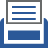printer friendly Reports: DNI656409-DNI6: Understanding Collisional Energy Transfer in Multi-Component Mixtures of Combustion Systems

Michael P. Burke, PhD, Columbia University

The overall conversion of fuel and oxygen to water and carbon dioxide during combustion proceeds through numerous individual reactions. A substantial fraction of these reactions are complex-forming (i.e. pressure-dependent) reactions, which depend on the collisional energy transfer characteristics of the surrounding mixture (“bath gas”).  In contrast to the pure-component bath gases used in nearly all fundamental investigations of these reactions, significant fractions of multiple species of disparate collisional energy transfer characteristics are present in combustion environments.  Further advancements in combustion modeling capabilities for realistic mixtures require a more comprehensive understanding of the way in which multi-component bath gases affect the collisional energy transfer in complex-forming reactions.

Our master equation studies have shown failures in conventional representations of multi-component mixture effects that reach an order of magnitude.  For example, Figure 1 shows deviations of the rate constant according to the conventional linear mixture rule, kLMR,P, from the rate constant calculated for the mixture using the master equation, k, for a prototypical single-well, multi-channel reaction.  In this multi-channel reaction, unimolecular decomposition can proceed through a lower energy tight transition state to form stable products or through a higher energy loose transition state to form two radicals.  The analysis was performed for a mixture of a weak collider and a strong collider. The results indicate that this deviation is strictly positive for the total decomposition rate constant and the rate constant for the lower energy channel.  However, the deviations for the higher energy channel can be positive or negative depending on the pressure.  These opposite directions of the deviations for the two channels significantly impact the branching ratio between formation of stable products or radicals, with clear implications for combustion radical chain reactions.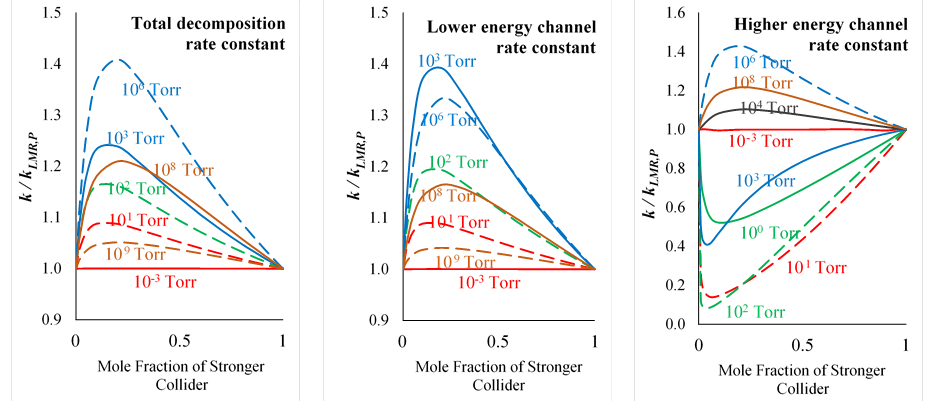Figure 1. Deviations of the rate constant according to the conventional linear mixture rule, kLMR,P, from the rate constant calculated for the mixture using the master equation, k.

We have developed improved mixture rules that account for the interactions between different colliders in their contributions to the rate constant (Table 1).  For example, our nonlinear mixture rule based on reduced pressure, kNMR,R, yields a nearly perfect replication of the mixture rate constant calculated in master equation calculations (Figure 2).  Instead of summing the rate constants for each collider evaluated at the same pressure (P) as in the conventional linear mixture rule, the nonlinear mixture rule involves a weighted sum of rate constants evaluated at the same reduced pressure (R).  The reduced pressure is a dimensionless parameter that compares the rate constants of the low-pressure limit, where energy transfer is infinitesimally slow, to the high-pressure limit, where the energy transfer is infinitely fast.  This normalization captures the fact that rate constants evaluated at the same reduced pressure for different colliders are much closer than those evaluated at the same pressure.  The weighting coefficient used for the weighted sum in the nonlinear mixture rule was derived through analytical solutions of the master equation in the low-pressure limit.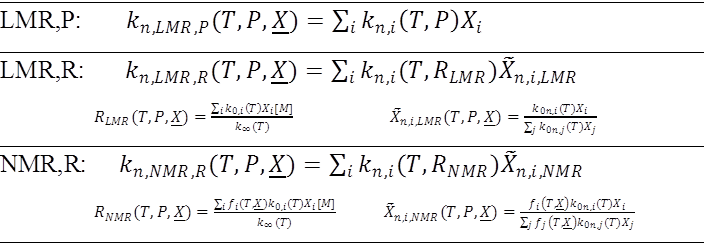Table 1. Various mixture rules for estimating rate constants in multi-component mixtures.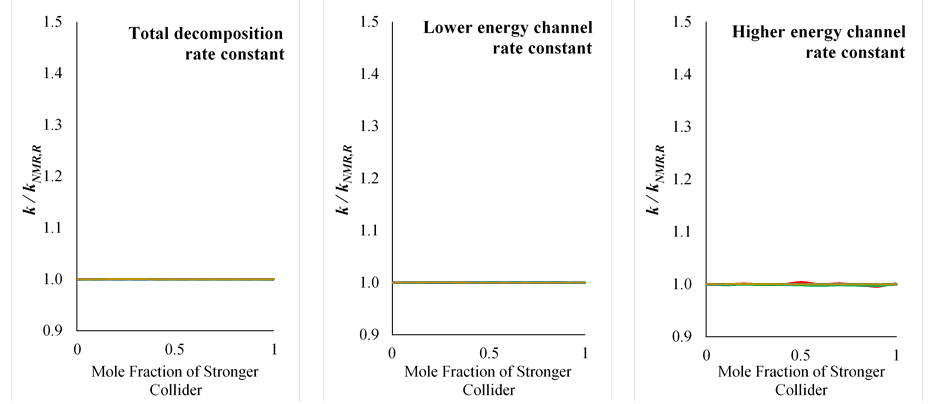Figure 2. Deviations of the rate constant according to our new nonlinear mixture rule, kNMR,R, from the rate constant calculated for the mixture using the master equation, k.

Another quintessential feature of multi-component combustion mixtures is the large fraction of reactive collision partners, which can induce bimolecular reactions on timescales that compete with energy transfer.  This can result in non-Boltzmann kinetic sequences in combustion mixtures where the energy from exothermic reactions is insufficiently randomized via collisional energy transfer prior to further reactions.  We have identified several non-Boltzmann kinetic sequences with important implications for combustion predictions, including for predictions of nitrogen oxide emissions formation as shown in Figure 3.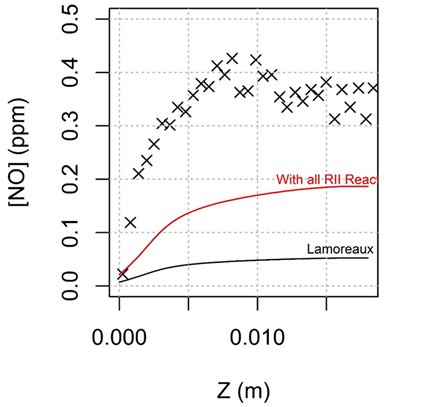Figure 3. Model predictions of nitrogen oxide, NO, in a burner stabilized flame using a kinetic model excluding non-Boltzmann reactions (black) and including non-Boltzmann reactions (red).

This Doctoral New Investigator grant from the ACS-PRF was my first research grant to be awarded.  It therefore is having a significant impact on my early career by helping me establish my research program at Columbia and advance computational methodologies for future investigations.  This grant has and is also contributing to the education and career development of the graduate students involved in this project.  The results we have generated during the first year of this project have been presented at numerous national and international conferences and we are in the process of preparing several manuscripts for archival journal publication.